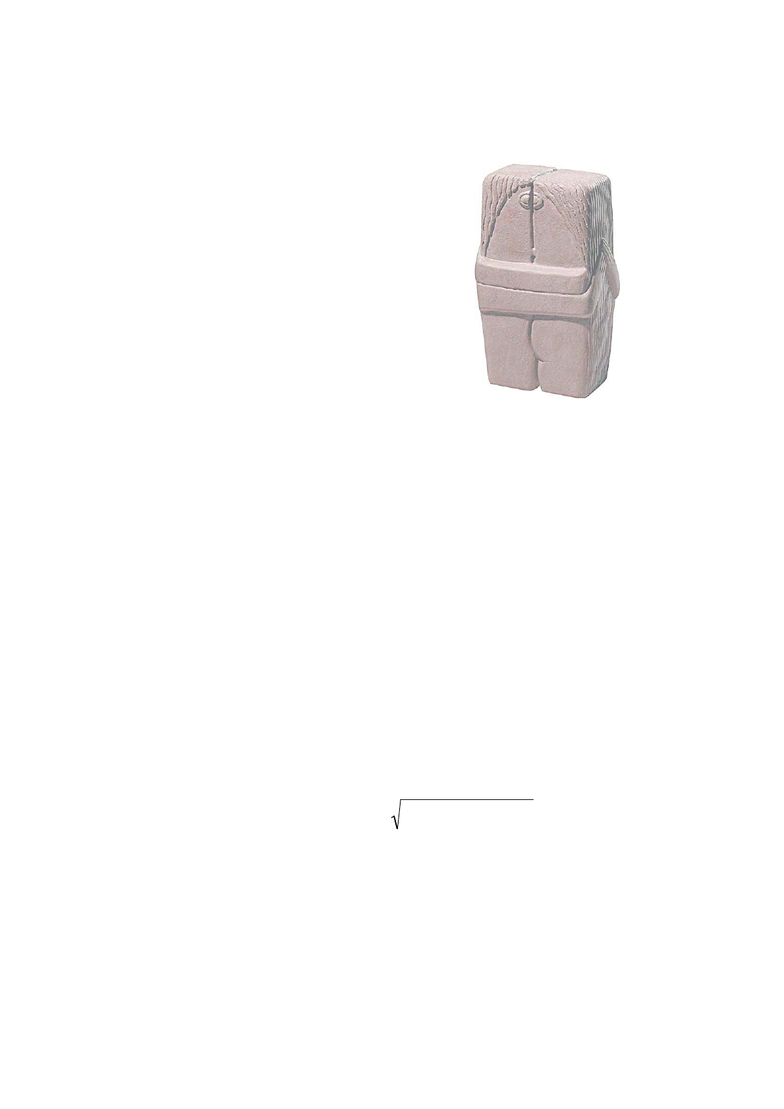# CS341 Lecture Notes - Closest Pair Of Points Problem, Euclidean Distance, Subroutine

27 views12 pages
School
University of Waterloo
Department
Computer Science
Course
CS3411
Closest Pair
and
Linear Time
Selection
The Kiss
by Brancusi
Closest Pair
Problem definition:
We are given npoints pi=(xi, yi), i = 1,, n.
How far apart are the closest pair of points?
We need the iand jvalues that minimize the
Euclidean distance:
Then return this distance.
Brute force: compute distances for all
n(n-1)/2pairs and find the minimum.
This algorithm runs in time Θ(n2).
xixj
( )2+(yiyj)2
Unlock document

This preview shows pages 1-3 of the document.
Unlock all 12 pages and 3 million more documents.2
Closest Pair by Divide and Conquer
Sort by x-coordinate, then:
• Divide:
Find vertical line splitting points in half.
• Conquer:
Recursively find closest pairs in each half.
Combine:
Check vertices near the border to see if any pair straddling
the border is closer together than the minimum seen so far.
Our goal:
Θ(n) overhead so that the total run time is Θ(n log n).
Implementation Details
Input: a set of points Psorted with respect to
the xcoordinate.
Initially, P= all points, and we pay Θ(n log n)
to sort them before making the first call to the
recursive subroutine.
Given the sorted points, it is easy to find the
dividing line.
Let PLbe points to the left of the dividing line.
Let PRbe points to the right of the dividing line.
Unlock document

This preview shows pages 1-3 of the document.
Unlock all 12 pages and 3 million more documents.3
Closest Pair Implementation Details
• Recursively:
Find closest pair in PL: Let
δ
Lbe their separation
distance
Similarly find closest pair in PR, with separation
distance
δ
R.
Clever observation: If the closest pair straddles the
dividing line, then each point of the pair must be
within
δ
= min{
δ
L,
δ
R} of the dividing line.
This will usually specify a fairly narrow band for our
Implementation Details
Suppose pand q, d(p,q)=
δ
are candidate closest points,
with pon the left and qon the
right of the dividing line.
qnot to the right of
δ
band.
if p= (x, y)then with ycoords
interval [y-
δ
, y+
δ
] can be
successfully paired with p.
So, we need only look at points
within
δ
above and below a
horizontal line through p.
Since the points in this rectangle
must be separated by at least δwe
have at most 6 points to
investigate. (Diagram shows this
“worst case” situation).
δ
δ
δ
p
Dividing Line Possible point q
Unlock document

This preview shows pages 1-3 of the document.
Unlock all 12 pages and 3 million more documents.